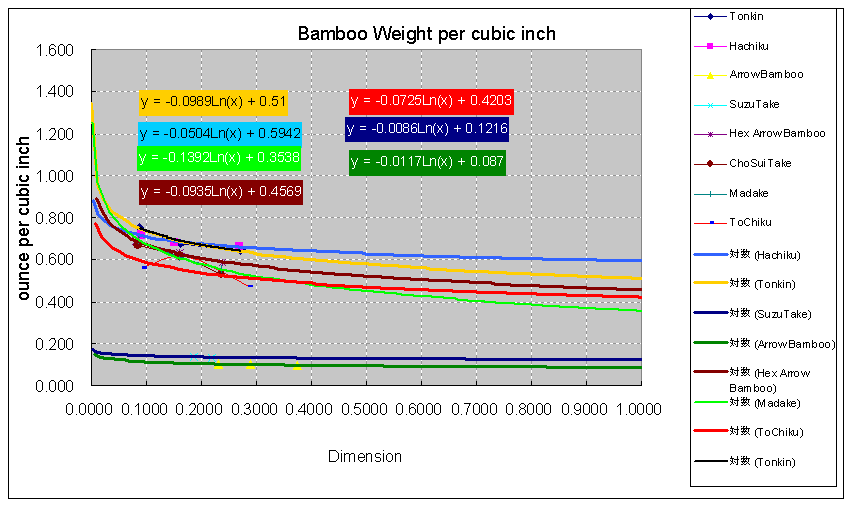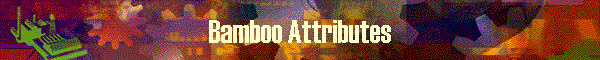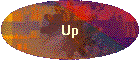This table is used in calculating moment value of rod blanks and  rod deflection.

Bamboo has different elasticity and weight by the specie, sometimes by the same culm of Tonkin.  So the selection of bamboo affects to the deflection and action of a resulted rod.

DynaRod can maintain attributes of elasticity and weight for several kind of bamboos in this table.  Of-course, user can make entries of materials for rod which are different from bamboo, such as wood, grass fiber, carbon graphite, even for the different side of the same Tonkin culm, etc..

After making the entry in this table, user can select one on combo box when specifying rod specification.

So far, several kind of bamboos are preset in the table which author investigated.  Even natural round bamboo shoot can also be utilized in DynaRod.

Tonkin (Chinese)
Hchiku (Japanese)
Arrow Bamboo (round, Hollowed)
Suzutake (Koyachiku, round thin)
Medake (Japanese, a thick Arrow Bamboo)
Cho-Sui-Chiku (Chinese)
Tohchiku (Garden Bamboo)

### Data on screen

Calculation function of MOE and Bamboo Weight/cubic inches

This function enables to know the MOE and bamboo weight which are used in calculation.
As the glued blank has different MOE and weight by the size of dimension, users need to
enter the dimension number as input and press [Calculate] button.

 Name Meaning Bamboo Name By selecting the entry of Bamboo Attribute Table via record selector, this field is auto filled. MOE value The calculated result of MOE is displayed Weight/ci The calculated result of Bamboo Weight per cubic inches is displayed. Dimension Input field of dimension number in either of inch or mm.

Function Button

 Name Function Reset clears out the displayed fields in calculation area. Calculate calculate and display MOE and Bamboo Weight/ci Close Closes this panel and return to the calling panel.

Bamboo Attribute Table contents

 Name Meaning Number Record id of table record Take Name Nick name of bamboo.  By this notation, user can select bamboo for a rod on spec. panels. L value The factor used to adjust the angle of bending in calculation.  Ordinarily set to 6.  Larger number will cause the bamboo to bend more. MOE K The K factor of MOE approximation graph. MOE=K x log (dimension) + C MOE C The C constant of MOE approximation graph. MOE=K x log (dimension) + C Weight K The K factor of bamboo weight approximation graph. Weight=K x log (dimension) + C Weight  C The C constant of bamboo weight approximation graph. Weight=K x log (dimension) + C Remarks comments

### Hybrid Rod

The table entry with TakeName="Hybrid" is recorded for the use of defining Hybrid/Hollow rod on specification panels.  Not an actual bamboo name.

Hybrid rod is the rod which uses different materials (bamboos) on each section and different specification of each section.  For instance, tip is made from softer bamboo Suzutke and butt is made from stiffer  Tonkin cane.  The Tip is hollowed and the butt is solid. Etc.

### How to define MOE (Modulus of Elasticity):

At first, we make a board like below;
gray: rectangular board,  light brown: wood block attached to board, brown: sample bamboo blank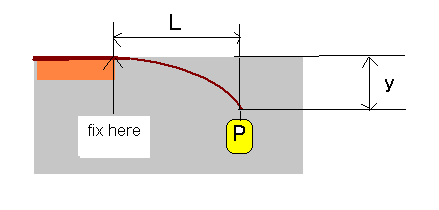Using a bamboo specie, we prepare three kind of sample blanks with different dimensions;

example:    large:  7mm (0.275in)   medium:    4mm (0.157in)    small:  1mm (0.0039in)

The length of samples are around;  30cm(11.8in)  for small and medium diameter and 70cm (17.55in) for large diameter sample.  Since the diameter and length are just used to calculate MOE, you can make them other appropriate number such as 0.3 inch instead of 0.275 inch, 0.004 inch for 0.0039 inch above.  The length are also all right by 1 feet for small and large diameter and 2 feet for large dimension sample.

The length from the edge of wood block to the end of the sample blank becomes (L).  Though the sample is bent in the picture above, (L) is the length for the straight sample blanks.

Set the sample like the picture above and hang some appropriate weight at the end of the sample, one by one.   Then measure the length of (y) from the top end of the board to know how much it got down.

Repeat this measurement for one sample blank by changing the weight with two to three kind of weight (e.g. 0.5 ounce and 1 ounce) and record all the trial for the values of; sample dimension, sample length(L), weight number(P) and for the sink (y).   To get the more accurate result, we may need the more sample weights.  We will average the results later.

Do this for all the sample blanks.

Now we have the experiment results for dimension (d), length (L), weight (P) and sink (y) for several different combinations.

For each sample blank (d), we will calculate MOE by the formula below;

y = PL^3 / 2 EI  where ^3 means power of 3

As we want to know E (MOE or young's ratio), we can modify the equation like below;

E = PL^3 / 2 yI

I (ai) is the factor called "Moment of Inertia" which is decided for the each cross section as follows;

Solid Hexagonal beam:  I = 0.5413 * b^4
where b is the length of one side of hexagon.   b=d/squareroute(3) where D is dimension

Hollowed Hexagonal beam:  I = 0.5413 * (b1^4 - b2^4)   where b1 is outer side length and b2 is inner (hole's) side length.

Solid Quad beam:  I = 1/12 * h^4  where h is the length of side (=dimension)

Hollowed Quad beam:  I = 1/12 * (h1^4 - h2^4)  where h1 is outer side length and h2 is inner side length.

Solid Round beam:  I = pai/64 * d^4  where d is diameter and equal to dimension in this case.

Hollowed Round beam:  I = pai/64 * (d1^4 -d2^4) where d1 is dimension and d2 is diameter of the hole. (inner diameter)

Next, we will use MS Excel graph function to know the MOE formula which is used in DynaRod.
Place the pairs of MOE number and sample dimension into the two columns of Excel sheet side by side.   Place all the experimented results here, for small, medium and large dimensions and for all the different weight numbers.
Using excel, we make x,y plot graph (scatter diagram) where x axis is for dimension in inch and y axis is for MOE value.   This set of plot represents one specie of bamboo.

Then, we will create average (or approximation) curve on this plot graph.  To do this, click the data on the displayed plot graph, then the data points are hi-lighted.  Then click right button of the mouse and select [add average (approximation) curve].

Then, a pop up window for the average (approximation) is displayed.
You will click [logarithm (O)] for approximation.
Next, open [option] tag and specify off for intercept and turn on check box for [display correlation formula].

Here, you can see a logarithmic graph and its formula of correlation as an averaged line.  We need this formula in order to set the values in DynaRod table.  These samples are shown on the graph below;  for example,  from the formula, y=-27421509(x)+23944786, we get -27421509 as the value of MOE K and 23944786 as MOE C.   You will set these number into the bamboo attribute table.

Similarly, you can set bamboo weight factors as Weight K and Weight C.

The following graphs shows MOE and bamboo weight per cubic inch.

MOE
*Sorry for some Japanese characters are displayed in the legend box.  Their meaning is approximation line by logarithm for each bamboo.  Please try to match by the color of the line.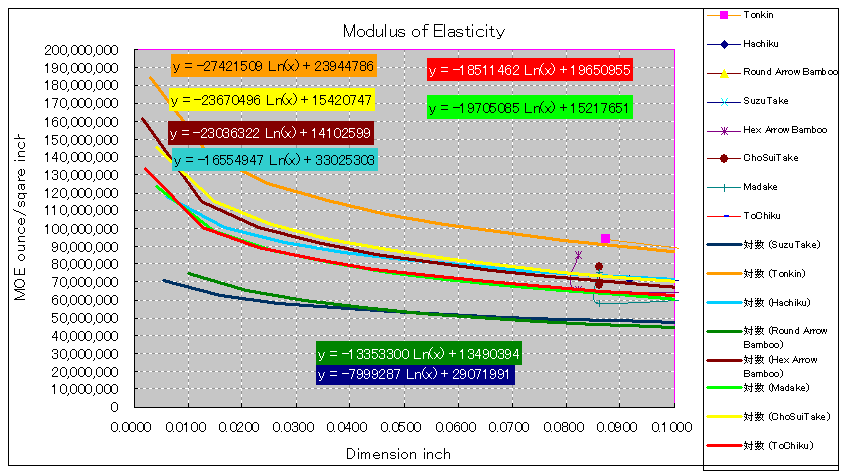### How to acquire L value (Deflection Adjustment factor)

The values of MOE or bamboo weight are the average of different pieces of actual bamboos.
To approximate the calculated results to the actual characters of bamboo ROD,  we are going to have a factor to adjust the variance.

Actual measurement: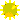Hold a completed bamboo rod horizontally of which taper is known.Fix  the rod just like the picture of the board above.Hang  a known weight at top guide of the rod.Measure how many inches the top guide falls down.

Calculation:Enter the taper of the measured (above) rod into DynaRodCalculate by [Deflection/Stress] (E) button, where Rod Type=Boat Rod.Enter the same weight value to the above into Weight field (or Fly weight field) on the (E) panel.Press [Calculate]When the calculation is completed, display [Graphs] panel and click [Excel/Zoom] button.Find a worksheet [Stress Data] and open it.Find the value at row=12, column=14 (point 0 on X axis since the worksheet is for vertical deflection).  This is the corresponding value to the measurement of above.Compare the measurement and the calculation and adjust L value on this table.Again, repeat the calculation and adjust L value until the calculated result gets near to the measurement.  Be sure the calculation is always "Approximation".

Bamboo Weight per cubic inch